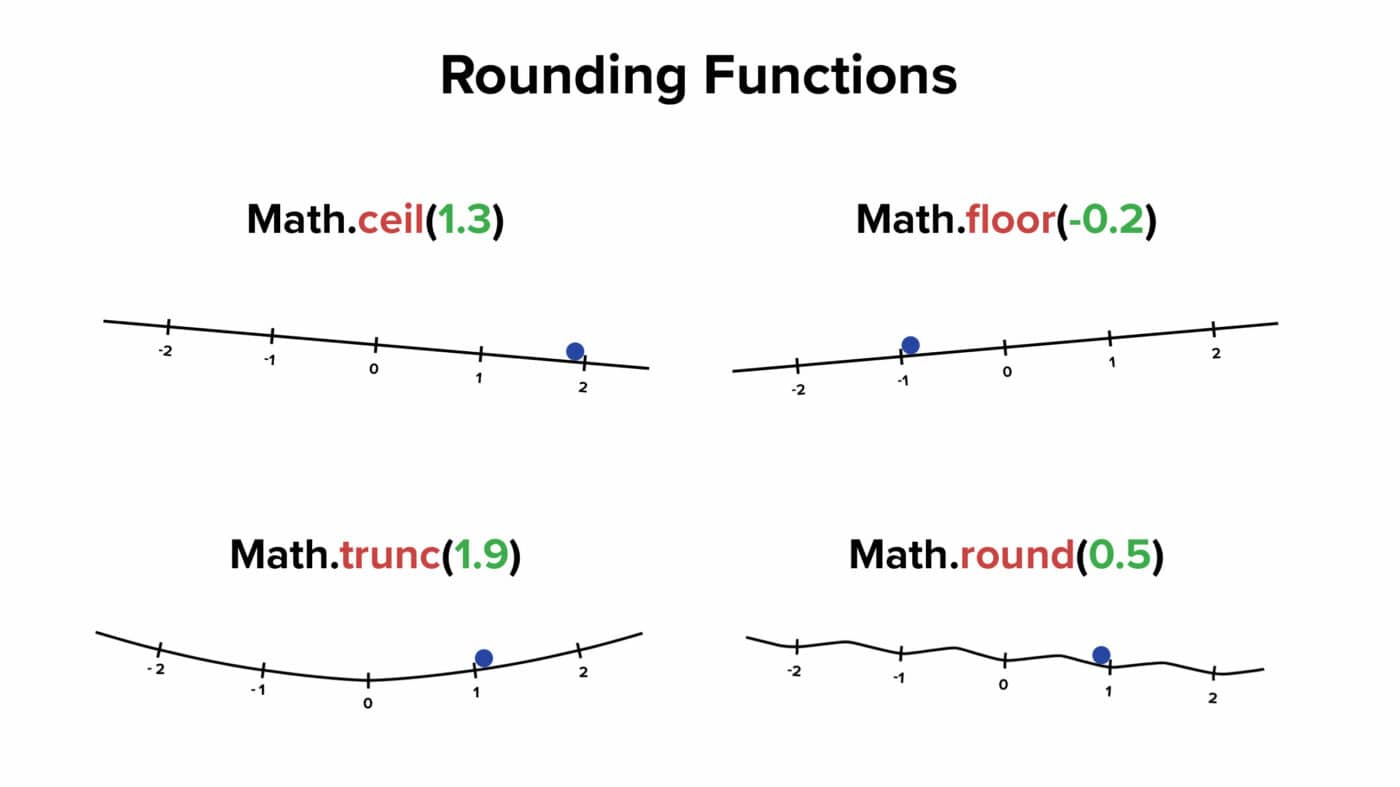# round

Learn how to use the round function in Notion formulas.

The `round()` function rounds its argument to the nearest integer (whole number).

```.wp-block-code {
border: 0;
}

.wp-block-code > div {
overflow: auto;
}

.shcb-language {
border: 0;
clip: rect(1px, 1px, 1px, 1px);
-webkit-clip-path: inset(50%);
clip-path: inset(50%);
height: 1px;
margin: -1px;
overflow: hidden;
position: absolute;
width: 1px;
word-wrap: normal;
word-break: normal;
}

.hljs {
box-sizing: border-box;
}

.hljs.shcb-code-table {
display: table;
width: 100%;
}

.hljs.shcb-code-table > .shcb-loc {
color: inherit;
display: table-row;
width: 100%;
}

.hljs.shcb-code-table .shcb-loc > span {
display: table-cell;
}

.wp-block-code code.hljs:not(.shcb-wrap-lines) {
white-space: pre;
}

.wp-block-code code.hljs.shcb-wrap-lines {
white-space: pre-wrap;
}

.hljs.shcb-line-numbers {
border-spacing: 0;
counter-reset: line;
}

.hljs.shcb-line-numbers > .shcb-loc {
counter-increment: line;
}

.hljs.shcb-line-numbers .shcb-loc > span {
}

.hljs.shcb-line-numbers .shcb-loc::before {
border-right: 1px solid #ddd;
content: counter(line);
display: table-cell;
text-align: right;
-webkit-user-select: none;
-moz-user-select: none;
-ms-user-select: none;
user-select: none;
white-space: nowrap;
width: 1%;
}```round(number)

number.(round)
``````

The exact midpoint between two whole numbers will round up towards positive infinity, not “away from zero”.

For example: `round(-4.5)` will round up to `4`.Note: Notion formulas don’t have a trunc() function (this graphic shows JavaScript rounding functions). But you can build your own trunc expression – see the bottom of this section in the ceil() article.
``````round(4.5) /* Output: 5 */

4.49.round() /* Output: 4 */

round(-4.49) /* Output: -4 */

-4.5.round() /* Output: -4 */

round(-4.51) /* Output: -5 */
``````

The `round()` function doesn’t accept additional arguments, so it can’t natively round a number to a specific decimal place.

However, you can accomplish this using the following formula:

``````/* Round to two decimal places */
round([number] * 100) / 100

/* Round to three decimal places */
round([number] * 1000) / 1000
``````

The number of zeroes corresponds to the number of decimal places to which your number will be rounded.

``````round(9.24551 * 10) / 10 /* Output: 9.2 */

(4.158015 * 100).round() / 100 /* Output: 4.16 */

round(5145.018394 * 10000) / 10000 /* Output: 5145.0184 */
``````

The example database below contains a formula that rounds the input number to several different decimal places.

``````"One Decimal: " + (round(prop("Number") * 10) / 10) +
"\nTwo Decimals: " + (round(prop("Number") * 100) / 100) +
"\nThree Decimals: " + (round(prop("Number") * 1000) / 1000) +
"\nFour Decimals: " + (round(prop("Number") * 10000) / 10000) +
"\nFive Decimals: " + (round(prop("Number") * 100000) / 100000)
``````

This formula demonstrates decimal-rounding to five different places.

Each rounded number is then converted to a string using the format function, allowing us to craft a five-line block of text.

Note how `\n` allows us to create a new line in the formula output.